# 11. Sorting Algorithms

This chapter discusses algorithms for sorting a set of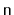items. This might seem like a strange topic for a book on data structures, but there are several good reasons for including it here. The most obvious reason is that two of these sorting algorithms (quicksort and heap-sort) are intimately related to two of the data structures we have already studied (random binary search trees and heaps, respectively).

The first part of this chapter discusses algorithms that sort using only comparisons and presents three algorithms that run in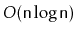time. As it turns out, all three algorithms are asymptotically optimal; no algorithm that uses only comparisons can avoid doing roughly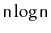comparisons in the worst-case and even the average-case.

Before continuing, we should note that any of the SSet or priority Queue implementations presented in previous chapters can also be used to obtain antime sorting algorithm. For example, we can sortitems by performing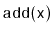operations followed by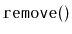operations on a BinaryHeap or MeldableHeap. Alternatively, we can useoperations on any of the binary search tree data structures and then perform an in-order traversal (Exercise 6.8) to extract the elements in sorted order. However, in both cases we go through a lot of overhead to build a structure that is never fully used. Sorting is such an important problem that it is worthwhile developing direct methods that are as fast, simple, and space-efficient as possible.

The second part of this chapter shows that, if we allow other operations besides comparisons, then all bets are off. Indeed, by using array-indexing, it is possible to sort a set ofintegers in the rangein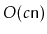time.

Subsections
opendatastructures.org